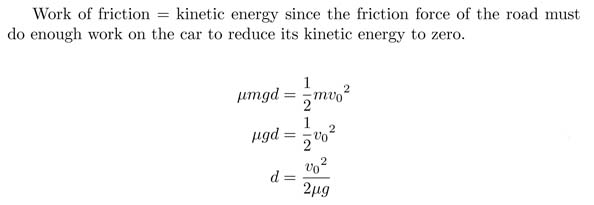SEARCH HOMEMath Central Quandaries & QueriesMr.Adjetey, a student: you are travelling at 30km/h. According to the rule of thumb,the braking distance is 9 metres when braking normally.How long is the braking distance under the same conditions when travelling at 60km/h?The long way to do this question is to solve for μ, the friction coefficient of the road, using 30 km/h information then solving for 60 km/h. The short way is to consider the formula for distance and think "If I double velocity, how will it effect distance?"

Hope this helps,

JaniceMath Central is supported by the University of Regina and The Pacific Institute for the Mathematical Sciences.